#### You may also like### Halving

These pictures show squares split into halves. Can you find other ways?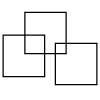### Three Squares

What is the greatest number of squares you can make by overlapping three squares?### Two Dice

Find all the numbers that can be made by adding the dots on two dice.

# Number Lines

## Number Lines

Leah and Tom both have number lines and a counter. They always start with their counters at zero.

Leah's number line goes along from left to right like this: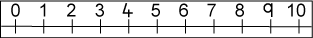First Leah made a jump of three along her number line and then a jump of four. Where did she land?

Next Leah made a secret jump along her number line. Then she made a jump of five and landed on $9$.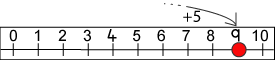How long was her secret jump?

Then Leah made a jump of six and another secret jump. She landed on $10$.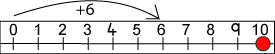How long was her second secret jump?

Tom's number line goes up and down like this: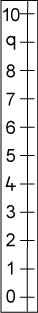First Tom made a jump of three up his number line and then a jump of two. Where did he land?

Next Tom made a secret jump up his number line. Then he made a jump of six and landed on $8$.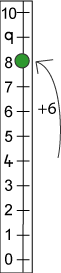How long was his secret jump?

Then Tom made a jump of four and another secret jump. He landed on $7$.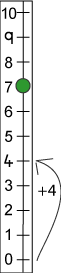How long was his second secret jump?

### Why do this problem?

This activity is a good one to try once children are familiar with number lines, and they have begun to use them for addition. It could be a good way to talk about subtraction.

The problem requires higher-order thinking compared with simply adding or subtracting. It encourages children to be confident in "undoing" operations, and therefore introduces them to the idea of subtraction being the inverse of addition and vice versa. However, you don't need to use this vocabulary at first.

The idea of having an unknown number (the secret jump) in a calculation (further jumps along the number line) is the essence of algebra, and this problem introduces that important concept but in an appropriate context.

### Possible approach

Start with a number line with which the children are familiar and then introduce a vertical number line if they have not met them before.

Involve the children in suggesting numbers to add using both number lines before introducing the idea of the 'secret jump'. Next, it might be appropriate to try some of your own examples with the group, asking them how they are going to find the secret number of jumps. Encourage pupils to explain how they will do it in their own words and discuss whether or not this will work.

Give children a counter and a number line to work on, either individually or in pairs. This sheet provides two number lines from $0$ - $10$, one horizontal and one vertical. It is intended to be cut into two and could be enlarged from A4 to A3. The children could then deal with the questions given in the problem and make up some examples to try on each other.

### Key questions

Are you starting or finishing with the secret jump?
Can you show me the jump on your number line?

### Possible extension

Use a number line which goes to up to $20$ or $100$.

### Possible support

Make sure that children are counting the jumps they make, and not the starting point. If there are difficulties with a vertical number line you could try this problem first.